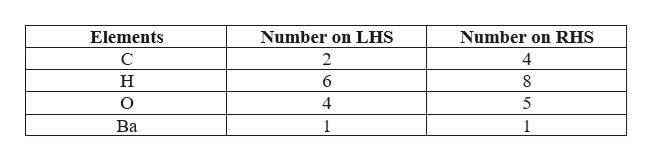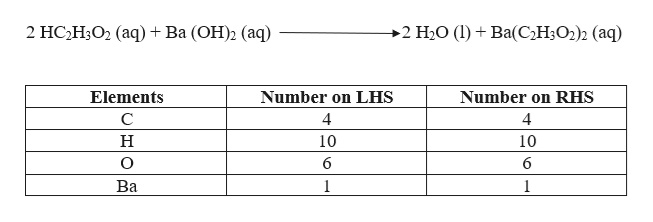# Consider the unbalanced equation for the neutralization of acetic acid:αHC2H3O2(aq)+βBa(OH)2(aq) →γH2O(l)+δBa(C2H3O2)2(aq)Balance the equation.Give your answer as an ordered set of numbers α, β, γ, δ. Your answers should reflect the lowest whole-number ratio of coefficients.

Question
56 views

Consider the unbalanced equation for the neutralization of acetic acid:

αHC2H3O2(aq)+βBa(OH)2(aq) →γH2O(l)+δBa(C2H3O2)2(aq)

Balance the equation.
Give your answer as an ordered set of numbers α, β, γ, δ. Your answers should reflect the lowest whole-number ratio of coefficients.
check_circle

Step 1

The given unbalanced equation is,

Step 2

To balance the chemical equation, the number of atoms on the left-hand side must be equal to number of atoms on the right side. The number of atoms in the unbalanced reaction is shown as,help_outlineImage TranscriptioncloseNumber on RHS Elements Number on LHS C 2 4 н 6 8 Ва 1 1 fullscreen
Step 3

To balance the above reaction, α = 2 and γ = 2. Substituting the values in the above equation, we ge...help_outlineImage Transcriptionclose2 HС НО2 (аq) + Ba (ОН)2 (аq) 2 H2O (1)Ba(C2H3O2)2 (aq) Elements Number on LHS Number on RHS C 4 4 н 10 10 6 6 Ва 1 1 fullscreen

### Want to see the full answer?

See Solution

#### Want to see this answer and more?

Solutions are written by subject experts who are available 24/7. Questions are typically answered within 1 hour.*

See Solution
*Response times may vary by subject and question.
Tagged in

### General Chemistry